## Representation of Data#

### Qubits#

A bit of data is represented by a single atom that is in one of two states denoted by $\ket0$ and $\ket1$. A single bit of this form is known as a qubit

A physical implementation of a qubit could use the two energy levels of an atom. An excited state representing $\ket1$ and a ground state representing $\ket0$.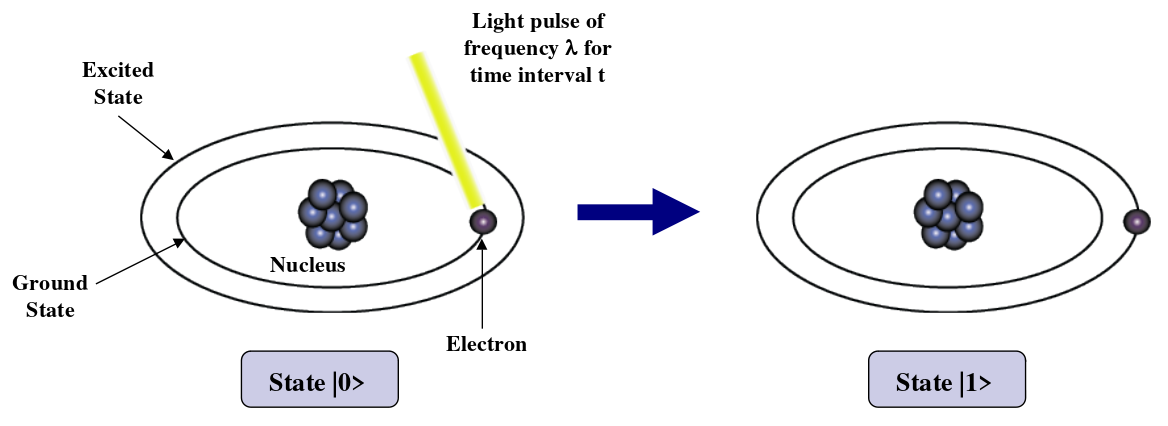### Superposition#

A single qubit can be forced into a superposition of the two states denoted by the addition of the state vectors:

$$\ket\psi=\alpha_1\ket0+\alpha_2\ket1$$

Where $\alpha_1$ and $\alpha_2$ are complex numbers and $|\alpha_1|^2+|\alpha_2|^2 = 1$

A qubit in superposition is in both of the states $\ket1$ and $\ket0$ at the same time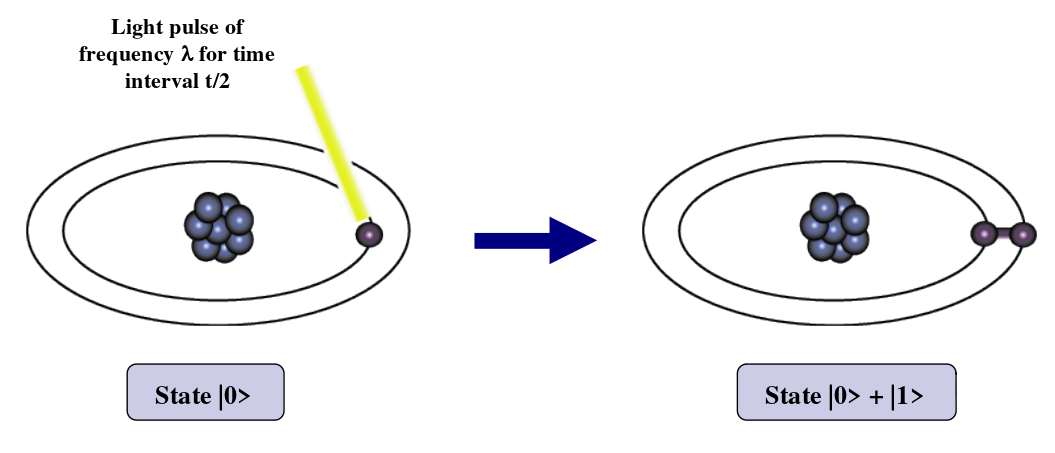Consider a 3 bit qubit register. An equally weighted superposition of all possible states would be denoted by:

$$\ket\psi = 1/\sqrt8\ket{000}+1/\sqrt8\ket{001}+…+1/\sqrt8\ket{111}$$

### Data Retrieval#

In general, an n qubit register can represent the numbers $0$ through $2^n-1$ simultaneously.

Sound too good to be true?…It is!

If we attempt to retrieve the values represented within a superposition, the superposition randomly collapses to represent just one of the original values.

In our equation: $\ket\psi=\alpha_1\ket0+\alpha_2\ket1$ , $\alpha_1$ represents the probability of the superposition collapsing to $\ket0$. The $\alpha_n$’s are called probability amplitudes. In a balanced superposition, $\alpha_n=1/\sqrt2$ where n is the number of qubits.

### Entanglement#

Entanglement is the ability of quantum systems to exhibit correlations between states within a superposition.

Imagine two qubits, each in the state $\ket0$ + $\ket1$ (a superposition of the 0 and 1.) We can entangle the two qubits such that the measurement of one qubit is always correlated to the measurement of the other qubit.

## Operations on Qubits#

### Reversible Logic#

Due to the nature of quantum physics, the destruction of information in a gate will cause heat to be evolved which can destroy the superposition of qubits.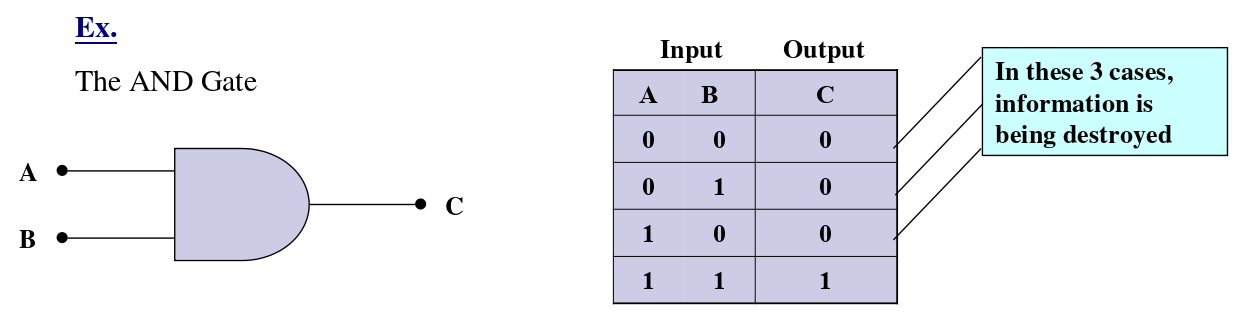This type of gate cannot be used. We must use Quantum Gates.

### Quantum Gates#

Quantum Gates are similar to classical gates, but do not have a degenerate output. i.e. their original input state can be derived from their output state, uniquely. They must be reversible.

This means that a deterministic computation can be performed on a quantum computer only if it is reversible. Luckily, it has been shown that any deterministic computation can be made reversible.(Charles Bennet, 1973)

Simplest gate involves one qubit and is called a Hadamard Gate (also known as a square-root of NOT gate.) Used to put qubits into superposition.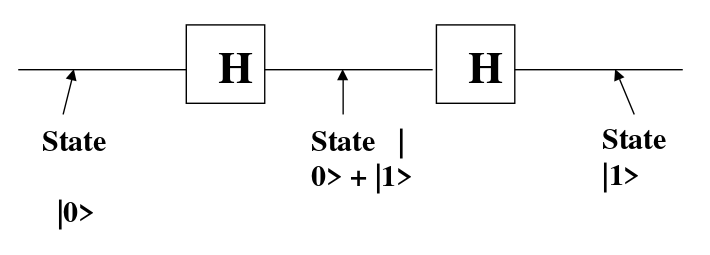Note: Two Hadamard gates used in succession can be used as a NOT gate

#### Controlled NOT#

A gate which operates on two qubits is called a Controlled-NOT (CN) Gate. If the bit on the control line is 1, invert the bit on the target line.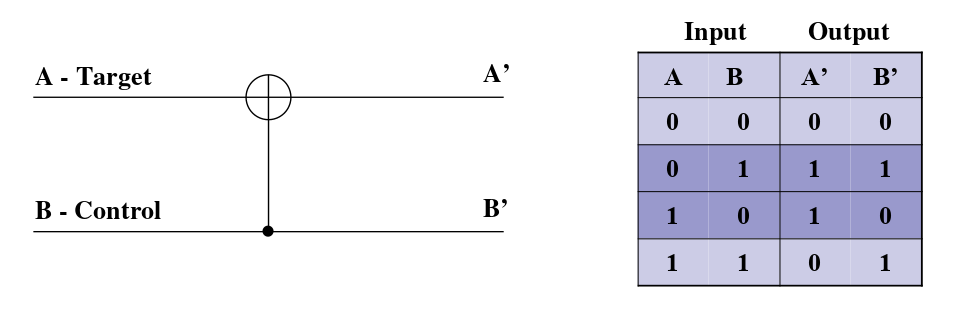Note: The CN gate has a similar behavior to the XOR gate with some extra information to make it reversible.

#### Multiplication By 2#

We can build a reversible logic circuit to calculate multiplication by 2 using CN gates arranged in the following manner: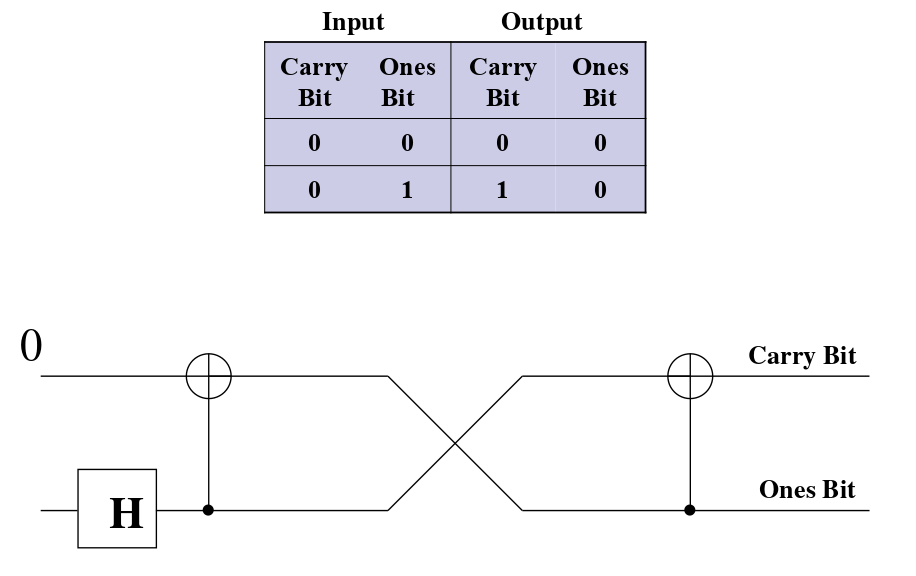#### Controlled Controlled NOT (CCN)#

A gate which operates on three qubits is called a Controlled Controlled NOT (CCN) Gate. Iff the bits on both of the control lines is 1,then the target bit is inverted.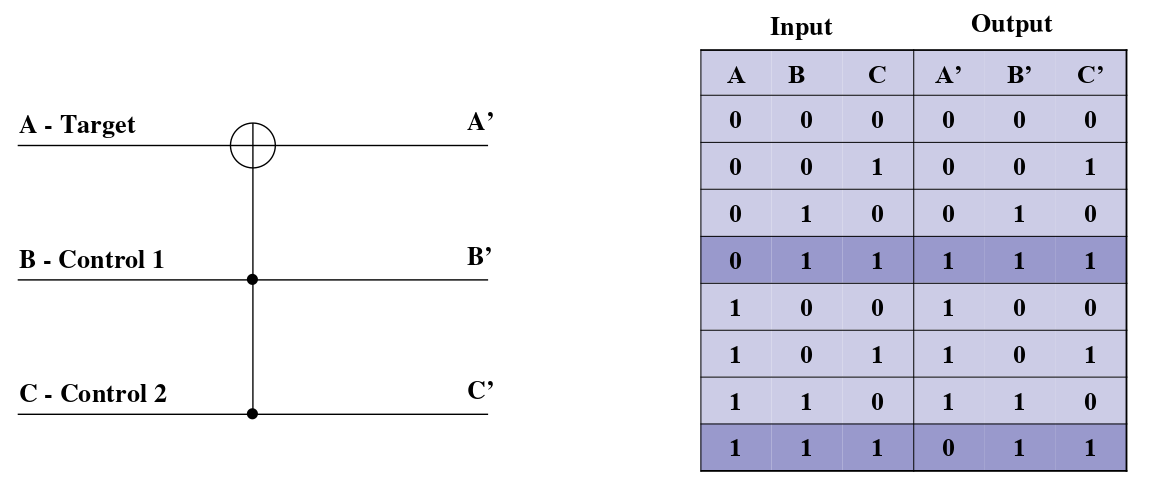### A Universal Quantum Computer#

The CCN gate has been shown to be a universal reversible logic gate as it can be used as a NAND gate.

CCN 门已被证明是一种通用的可逆逻辑门，因为它可以用作与非门。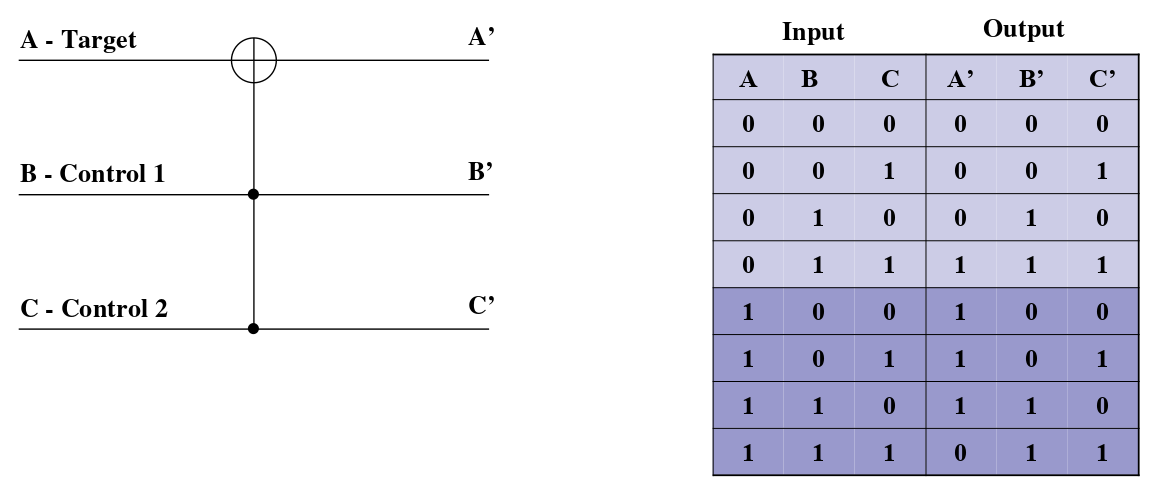When our target input is 1, our target output is a result of a NAND of B and C.

## Reference#

• Joseph Stelmach, Quantum Computer

https://www.eecis.udel.edu/~saunders/courses/879-03s/quantumComputers.ppt

• wikipedia, probability amplitudes

https://en.wikipedia.org/wiki/Probability_amplitude

• wikipedia, Quantum superposition

https://en.wikipedia.org/wiki/Quantum_superposition